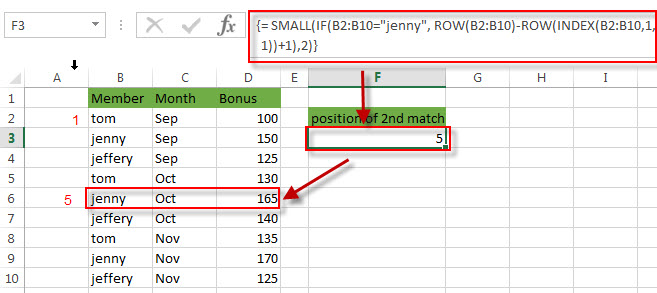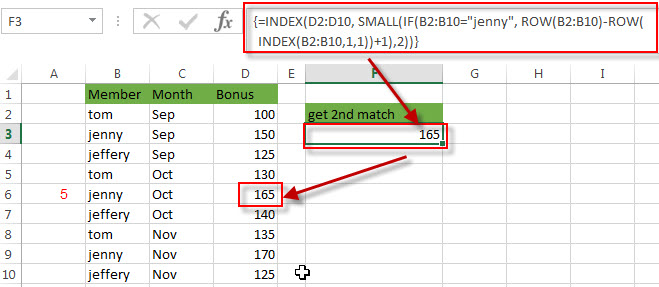# How to get nth Match with One Criteria using INDEX/MATCH

This post will guide you how to get nth match based on one criteria with INDEX and MATCH in Excel. In the previous post, we used one excel formula to get the position of the nth occurrence of a value based on one criteria, at this moment, we can also use it in the new formula to extract the nth match value using INDEX function. Like the below array formula:

`{=INDEX(array,SMALL(IF(vals=val,ROW(vals)-ROW(INDEX(vals,1,1))+1),nth))}`

For example, if you want to find the 2th occurrence of the member “jenny” in the range B2:B10 and extracts its relative bonus value in the range D2:D10, you can used the following array formula:

`=INDEX(D2:D10, SMALL(IF(B2:B10="jenny", ROW(B2:B10)-ROW(INDEX(B2:B10,1,1))+1),2))`

Let’s see how this formula works

= SMALL(IF(B2:B10=”jenny”, ROW(B2:B10)-ROW(INDEX(B2:B10,1,1))+1),2)The detailed description for this formula, please continue reading: get the position of nth occurrence of a value in column

The SMALL function returns the position of the second occurrence of the string text “jenny” in the range B2:B10. If you want to get the third or nth match value in a range, just need to modify the nth in the formula as your need.

=INDEX(D2:D10, SMALL(IF(B2:B10=”jenny”, ROW(B2:B10)-ROW(INDEX(B2:B10,1,1))+1),2))The First INDEX function returns bonus value of the second match based on the position number returned by the SMALL function. So it returns \$165.

### Related Formulas

• Find nth Occurrence with Multiple Criteria Using INDEX/MATCH
If you want to find the nth occurrence with multiple criteria, you can use a combination with the INDEX function, SMALL function, nested IF function and ROW function to create a complex excel formula like this:=INDEX(Array,SMALL(IF(Range1…
• Reverse a List or Range
If you want to reverse a list or range, you can use a combination of the INDEX function, the COUNTA function, the ROW function or ROWS function to create a new formula. you can use the following formula:=INDEX(\$A\$2:\$A\$5,COUNTA(\$A\$2:\$A\$5)-ROWS(\$C\$2:C2)+1)…
• Transpose Values Based on the Multiple Lookup Criteria
If you want to lookup the value with multiple criteria, and then transpose the last results, you can use the INDEX function with the MATCH function to create a new formula.…
• Lookup the Value with Multiple Criteria
If you want to lookup the value with multiple criteria in a range, you can use a combination with the INDEX function and MATCH function to create an array formula.…
• Lookup the Next Largest Value
If you want to get the next largest value in another column, you can use a combination of the INDEX function and the MATCH function to create an excel formula..

### Related Functions

• Excel INDEX function
The Excel INDEX function returns a value from a table based on the index (row number and column number)The INDEX function is a build-in function in Microsoft Excel and it is categorized as a Lookup and Reference Function.The syntax of the INDEX function is as below:= INDEX (array, row_num,[column_num])…
• Excel MATCH function
The Excel MATCH function search a value in an array and returns the position of that item.The syntax of the MATCH function is as below:= MATCH  (lookup_value, lookup_array, [match_type])….
• Excel SMALL function
The Excel SMALL function returns the smallest numeric value from the numbers that you provided. Or returns the smallest value in the array.The syntax of the SMALL function is as below:=SMALL(array,nth) …
• Excel ROW function
The Excel ROW function returns the row number of a cell reference.The ROW function is a build-in function in Microsoft Excel and it is categorized as a Lookup and Reference Function.The syntax of the ROW function is as below:= ROW ([reference])….
• Excel IF function
The Excel IF function perform a logical test to return one value if the condition is TRUE and return another value if the condition is FALSE. The IF function is a build-in function in Microsoft Excel and it is categorized as a Logical Function.The syntax of the IF function is as below:= IF (condition, [true_value], [false_value])….
Related Posts

Extract matching values From Two Lists

Suppose that you are working with two lists containing few values, and you want to extract the matching values from those two lists into another separate list. You might prefer to manually extract the matching values from the two lists, ...

Extract all Partial Matches

Just assume that you have a range of data that contains a text string and you want to extract the few partial matches into another separate range of cells; then you might think that it's not a big deal; because ...

Extract Multiple Match Values into Separate Columns

If you have a few values/items in the excel sheet and you are thinking that with the aid of the “VlOOKUP” function you can look for a specific value, extract it and then put the matching item into the separate ...

How to Sum the Smallest N Values in Excel

Sometimes we may want to sum the first smallest N numbers in a range in Excel. In this article, we will show you the method of “SUM the Smallest N Numbers” by a simple formula which consist of SUMPRODUCT and ...

How to Create Dynamical Drop-Down List and Sort by Alphabetical Order in Excel

In our daily work we may need to create a dynamical dropdown list and sort all values by alphabetical order. To create a dropdown list like this, we need to apply some built-in features like ‘Define Name’ and ‘Data Validation’ ...

Sidebar